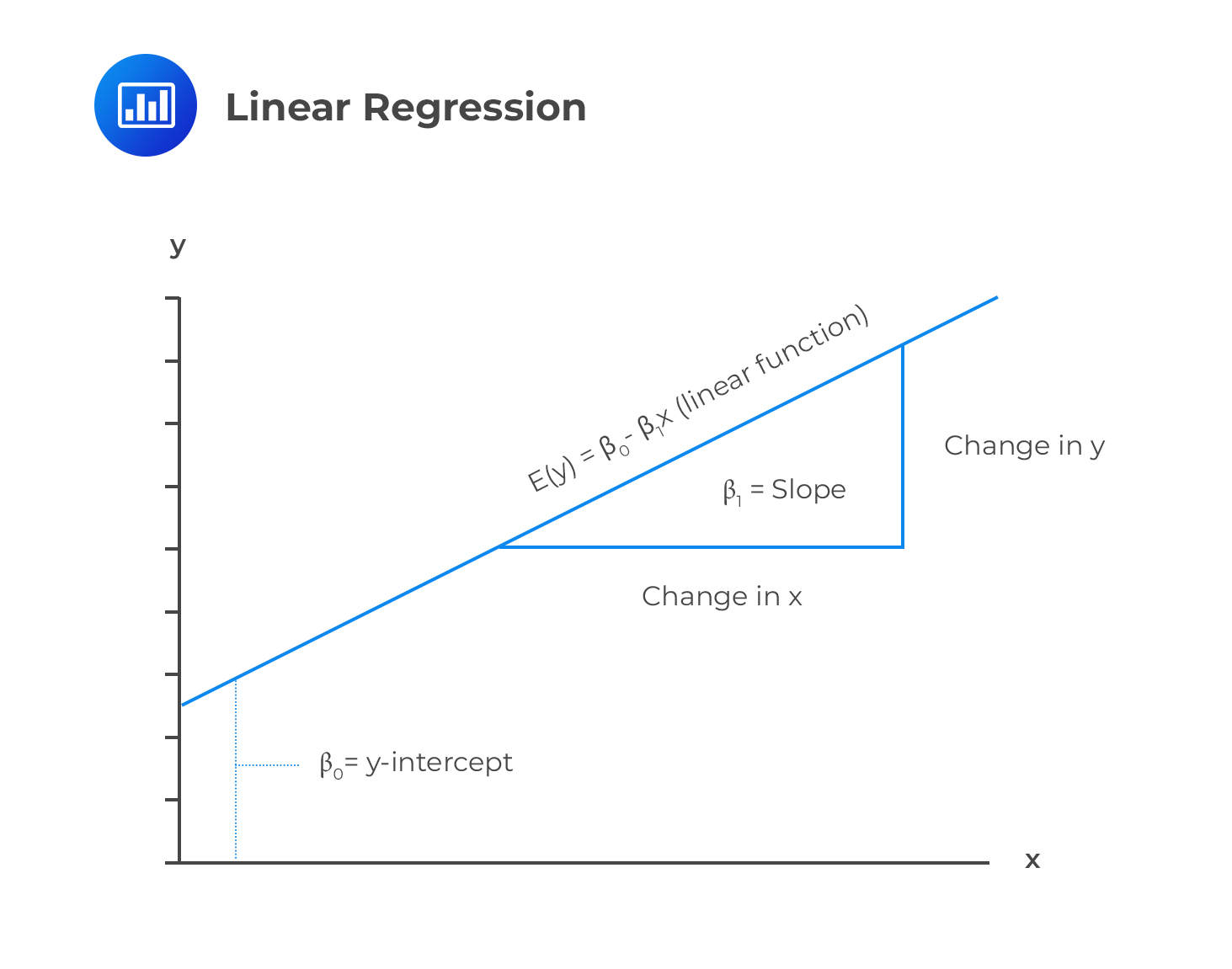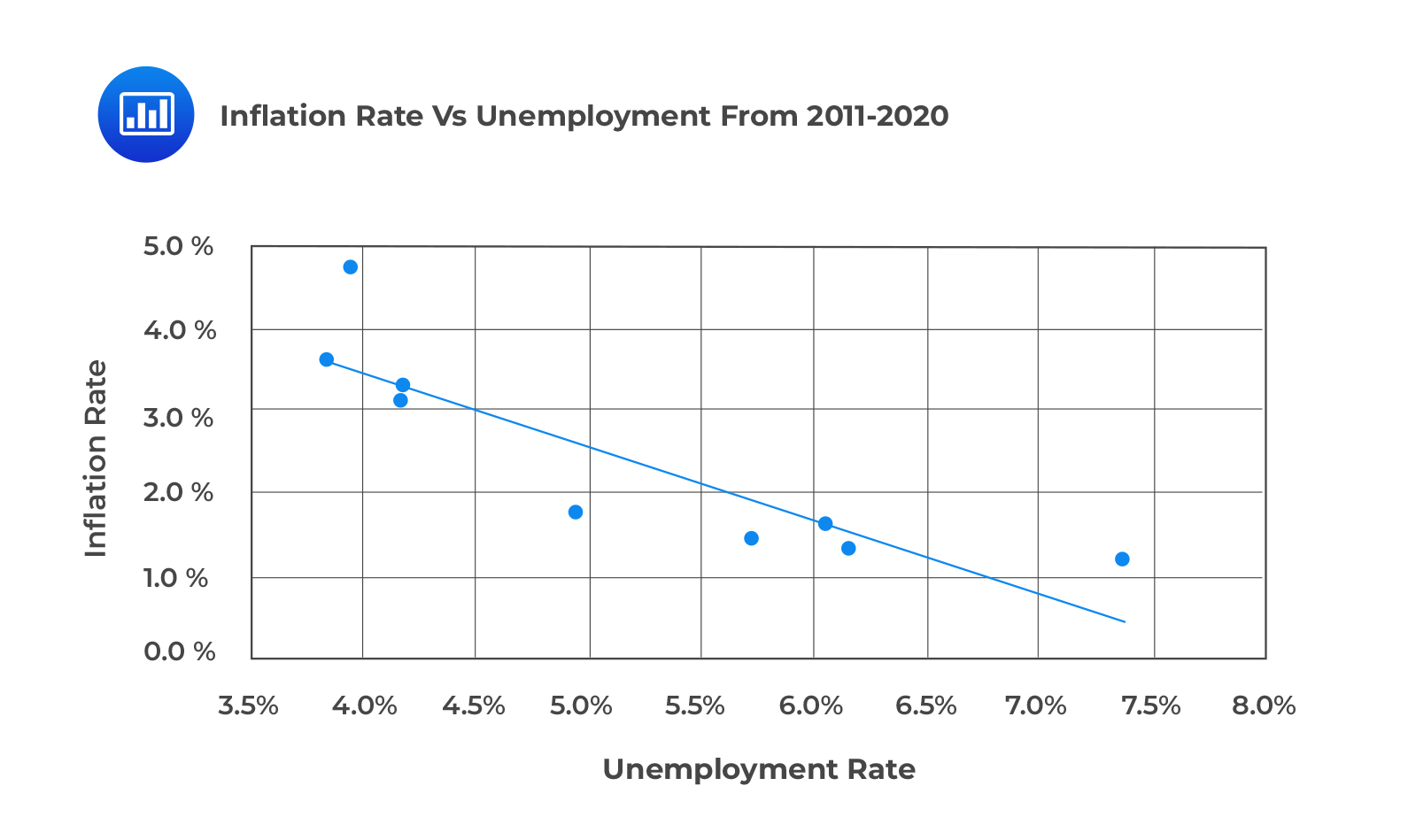# Dependent and Independent Variables

Linear regression forecasts the value of a dependent variable given the value of an independent variable. It assumes that there is a linear relationship between dependent and independent variables.

A dependent variable is predicted by an independent variable and is also known as the explained variable or endogenous variable.  An independent variable, on the other hand, explains the variation in the dependent variable. It is also known as the exogenous variable, explanatory variable, or predicting variable.

The following is a simple linear regression equation:

$$Y=\beta_0 +\beta_1X_1+\epsilon$$

Where:

$$Y$$ = dependent variable.

$$\beta_0$$ = intercept.

$$\beta_1$$ = slope coefficient.

$$X$$ = independent variable.

$$\epsilon$$ = error term (Noise).

$$\beta_0$$ and $$\beta_1$$ are known as regression coefficients.

The error term refers to the part of the dependent variable that cannot be explained by the independent variable.

Below is an illustration of a simple linear regression model.### Example: Dependent and Independent Variables

Arth Shah is using regression analysis to forecast inflation given unemployment data from 2011 to 2020.

The following table shows the relevant data from 2011 to 2020.

$$\small{\begin{array}{c|c|c}\textbf{Year} & \textbf{Unemployment Rate} & \textbf{Inflation rate}\\ \hline 2011 & 6.1\% & 1.7\%\\ \hline 2012 & 7.4\% & 1.2\%\\ \hline 2013 & 6.2\% & 1.3\%\\ \hline 2014 & 6.2\% & 1.3\%\\ \hline 2015 & 5.7\% & 1.4\%\\ \hline 2016 & 5.0\% & 1.8\%\\ \hline 2017 & 4.2\% & 3.3\%\\ \hline 2018 & 4.2\% & 3.1\%\\ \hline 2019 & 4.0\% & 4.7\%\\ \hline 2020 & 3.9\% & 3.6\%\\ \end{array}}$$

A scatter plot of the inflation rates against unemployment rates from 2011 to 2020 is shown in the following figure.What variable is the dependent variable?

#### Solution

Inflation is the dependent variable: It is the variable predicted using the unemployment rates.

## Question

The independent variable in a regression model is most likely the:

A. predicted variable.

B. predicting variable.

C. endogenous variable.

### Solution

An independent variable explains the variation of the dependent variable. It is also called the explanatory variable, exogeneous variable, or the predicting variable.

A and C are incorrect. A dependent variable is a variable predicted by the independent variable. It is also known as the predicted variable, explained variable, or the endogenous variable.

Shop CFA® Exam Prep

Offered by AnalystPrepLevel I
Level II
Level III
All Three Levels
Featured Shop FRM® Exam PrepFRM Part I
FRM Part II
FRM Part I & Part II
Learn with Us

Subscribe to our newsletter and keep up with the latest and greatest tips for success
Shop Actuarial Exams PrepExam P (Probability)
Exam FM (Financial Mathematics)
Exams P & FM
Shop GMAT® Exam PrepComplete CourseSergio Torrico
2021-07-23
Excelente para el FRM 2 Escribo esta revisión en español para los hispanohablantes, soy de Bolivia, y utilicé AnalystPrep para dudas y consultas sobre mi preparación para el FRM nivel 2 (lo tomé una sola vez y aprobé muy bien), siempre tuve un soporte claro, directo y rápido, el material sale rápido cuando hay cambios en el temario de GARP, y los ejercicios y exámenes son muy útiles para practicar.diana
2021-07-17
So helpful. I have been using the videos to prepare for the CFA Level II exam. The videos signpost the reading contents, explain the concepts and provide additional context for specific concepts. The fun light-hearted analogies are also a welcome break to some very dry content. I usually watch the videos before going into more in-depth reading and they are a good way to avoid being overwhelmed by the sheer volume of content when you look at the readings.Kriti Dhawan
2021-07-16
A great curriculum provider. James sir explains the concept so well that rather than memorising it, you tend to intuitively understand and absorb them. Thank you ! Grateful I saw this at the right time for my CFA prep.nikhil kumar
2021-06-28
Very well explained and gives a great insight about topics in a very short time. Glad to have found Professor Forjan's lectures.Marwan
2021-06-22
Great support throughout the course by the team, did not feel neglectedBenjamin anonymous
2021-05-10
I loved using AnalystPrep for FRM. QBank is huge, videos are great. Would recommend to a friendDaniel Glyn
2021-03-24
I have finished my FRM1 thanks to AnalystPrep. And now using AnalystPrep for my FRM2 preparation. Professor Forjan is brilliant. He gives such good explanations and analogies. And more than anything makes learning fun. A big thank you to Analystprep and Professor Forjan. 5 stars all the way!michael walshe
2021-03-18
Professor James' videos are excellent for understanding the underlying theories behind financial engineering / financial analysis. The AnalystPrep videos were better than any of the others that I searched through on YouTube for providing a clear explanation of some concepts, such as Portfolio theory, CAPM, and Arbitrage Pricing theory. Watching these cleared up many of the unclarities I had in my head. Highly recommended.